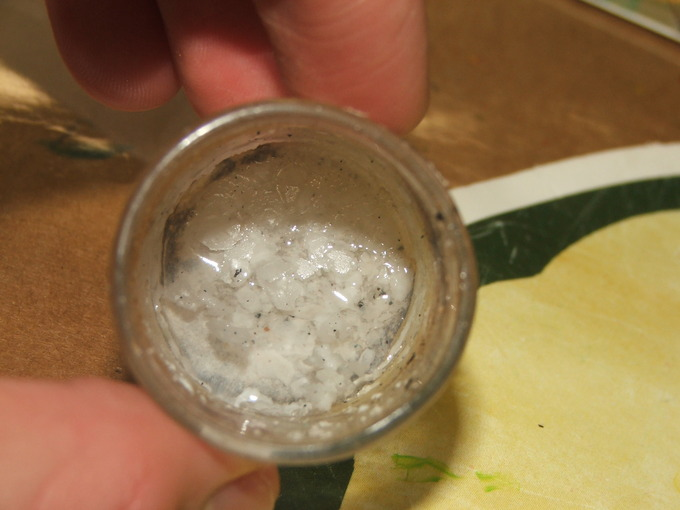## Effect of a Common Ion on Solubility

#### Learning Objective

• Calculate the molar solubility of a compound in solution containing a common ion.

#### Key Points

• Adding a common ion decreases the solubility of a solute.
• The common-ion effect can be used to separate compounds or remove impurities from a mixture.
• Different common ions have different effects on the solubility of a solute based on the stoichiometry of the balanced equation.

#### Terms

• precipitateA solid that exits the liquid phase of a solution.
• precipitateTo come out of a liquid solution into solid form.
• limestoneAn abundant rock of marine and fresh-water sediments; primarily composed of calcite (CaCO₃); it occurs in a variety of forms, both crystalline and amorphous

## Adding a Common Ion

If you have a solution and solute in equilibrium, adding a common ion (an ion that is common with the dissolving solid) decreases the solubility of the solute. This is because Le Chatelier’s principle states the reaction will shift toward the left (toward the reactants) to relieve the stress of the excess product. When equilibrium is shifted toward the reactants, the solute precipitates.Lithium hydroxide with carbonate growthsLithium hydroxide forms less-soluble lithium carbonate, which precipitates because of the common ion effect.

Scientists take advantage of this property when purifying water. In areas where water sources are high in chalk or limestone, drinking water contains excess calcium carbonate CaCO3. In the water treatment process, sodium carbonate salt is added to precipitate the calcium carbonate. The very pure and finely divided precipitate of calcium carbonate that is generated is used in the manufacture of toothpaste.

## Example:

What is the solubility at 25°C of calcium fluoride (CaF2): (a) in pure water; (b) in 0.10 M calcium chloride (CaCl2); and (c) in 0.10 M sodium fluoride (NaF)?

Solution:

$CaF_2 \leftrightarrow Ca^{2+} + 2F^-$

(a) If the solubility in pure water is s, then

[Ca2+] = s and [F] = 2s

$K_{sp} = {[Ca^{2+}]}{[F^-]}^2$

Ksp = s(2s)2 = 4s3 = 3.9 x 10-11

s = 2.1 x 10-4 M

(b) Here the calcium ion concentration is the sum of the concentrations of calcium ions from the 0.10 M calcium chloride and from the calcium fluoride whose solubility we are seeking:

[Ca2+] = 0.10 + s

[F] = 2s

Ksp = (0.10 + s)(2s)2 = 3.9 x 10-11

Can we simplify this equation? With such a small solubility product for CaF2, you can predict its solubility << 0.10 moles per liter. If our prediction is valid, we can simplify the solubility-product equation:

Ksp = 0.10 (2s)2 = 0.40s2 = 3.9 x 10-11

s2 = $\frac{3.90 \times 10^{-11}}{0.40}$ = 9.75 x 10-11

s = 9.9 x 10-6 M

If we go back and compare, only 4.7 percent as much CaF2 will dissolve in 0.10 M CaCl2 as in pure water:

$\frac{(9.9 \times 10^{-6})}{2.1 \times 10^{-4}}$ x 100 = 4.7%

Therefore, the approximation that s is small compared to 0.10 M was reasonable.

(c) In 0.10 M NaF:

[Ca2+] = s

[F] = 0.10 + 2s

since fluoride ions are in NaF as well as in CaF2.

The solubility-product equation is:

Ksp = s(2s + 0.10)2 = 3.9 x 10-11

Again, the equation can be simplified. The 2s term is << 0.10 moles per liter, and therefore:

Ksp = s(0.10)2 = 3.9 x 10-11

s = 3.9 x 10-9 M

This approximation is also valid, since only 0.0019 percent as much CaF2 will dissolve in 0.10 M NaF as in pure water. Fluoride is more effective than calcium as a common ion because it has a second-power effect on the solubility equilibrium.